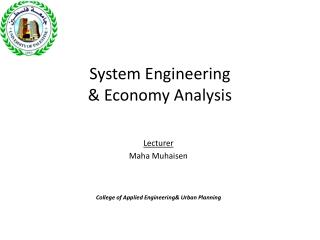Download PresentationSystem Engineering & Economy Analysis

# System Engineering & Economy Analysis - PowerPoint PPT PresentationDownload Presentation## System Engineering & Economy Analysis

- - - - - - - - - - - - - - - - - - - - - - - - - - - E N D - - - - - - - - - - - - - - - - - - - - - - - - - - -
##### Presentation Transcript

1. System Engineering & Economy Analysis Lecturer Maha Muhaisen College ofApplied Engineering& Urban Planning

2. Discussion • Chapter (1)

3. Q-27 • A farmer must decide what combination of seed, water, fertilizer, and pest control will be most profitable, for the coming year. The local agricultural college did a study of this farmer's situation and • prepared the following table. Plan Cost/acre income/acre A \$600 \$ 800 B 1500 1900 C 1800 2250 D 2100 2509 • the last page of the college's study was torn off, and hence the farmer is not sure which plan the agricultural college recommends. Which plan should the farmer adopt? Explain.

4. Solution Plan Cost/acre income/acre Profit A \$600 \$ 800 \$200 B 1500 1900 400 C 1800 2250 450 D 2100 2509 400 To maximize profit, choose alternative C.

5. Q-33 • Marie., a college student, is getting ready for three final examination at the end of the school year. Between now and the start of exams, she has 15 hours of study time available. She would like to get as high a grade average as possible in her math, physics, and engineering economy classes. • She feels she must study at least 2 hours for each course and if necessary, will settle for low grade that limited study would yield. • How much time should Maria devote to each class if she estimate her grade in each subject as follows:

6. Solution • The question here is how to apportion the available 15 hrs of study among the courses. One might begin for example, assuming 5hrs of study for each course. The combined total score would be 190. • Further study would show that the best use of time is : • Math 4hrs 44 • Physics 7 hrs 77 • Eng. Economy 4 hrs 71 • Total 192 hrs

7. Q-31 • On her first engineering job, Joy Hayes was given the responsibility of determining the production rate for a new product. She has assembled data as indicated • (a) Select an appropriate economic criterion and estimate-the production rate based upon it • Joy's boss told Joy: "I want you to maximize output with minimum input, Joy wonders if it is possible to achieve her boss's criterion. • She asks your advice. What would you tell her?

8. solution • The suitable criterion is to maximize net profit • The date from graphs may be tabulated as follows: • The best production rate is 150 unit/hr • B. minimum input is zero & max. output is 250 units/hr. since one can not achieve max. output at minimum input, the statement makes no sense.

9. Q. 40 • a firm is planning t manufacture a new product. The sales dep. estimates that the quantity that can be sold deponds on the selling price. As the selling price is increased, the quantity that can be sold decreases, numerically they estimate: • P = \$ 35 – 0.02 Q • Where P = selling price per unit. • Q = quantity sold per year. • On the other hand, the management estimates that the average cost of manufacturing and selling the product will decrease as the quantity sold increases. They estimate • C = \$ 4Q - \$ 8000, where C = cost to product and sell Q per year.

10. The firm’s management wishes to produce and sell the product at rate that will be a maximum. What quantity should the decision makers plan to produce and sell each year? • Solution : Profit = income – cost = PQ – C , where, PQ = 35Q – 0.02Q2 C = 4Q +8000 Profit = 35Q – 0.02Q2+-4Q-8000 = 31Q – 0.02Q2 – 8000  D (profit) / dq = 31 – 0.04Q solve for Q  Q = 775 units /year

11. Solution : Profit = income – cost = PQ – C , where, PQ = 35Q – 0.02Q2 C = 4Q +8000  Profit = 35Q – 0.02Q2+-4Q-8000 = 31Q – 0.02Q2 – 8000  D (profit) / dq = 31 – 0.04Q solve for Q  Q = 775 units /year